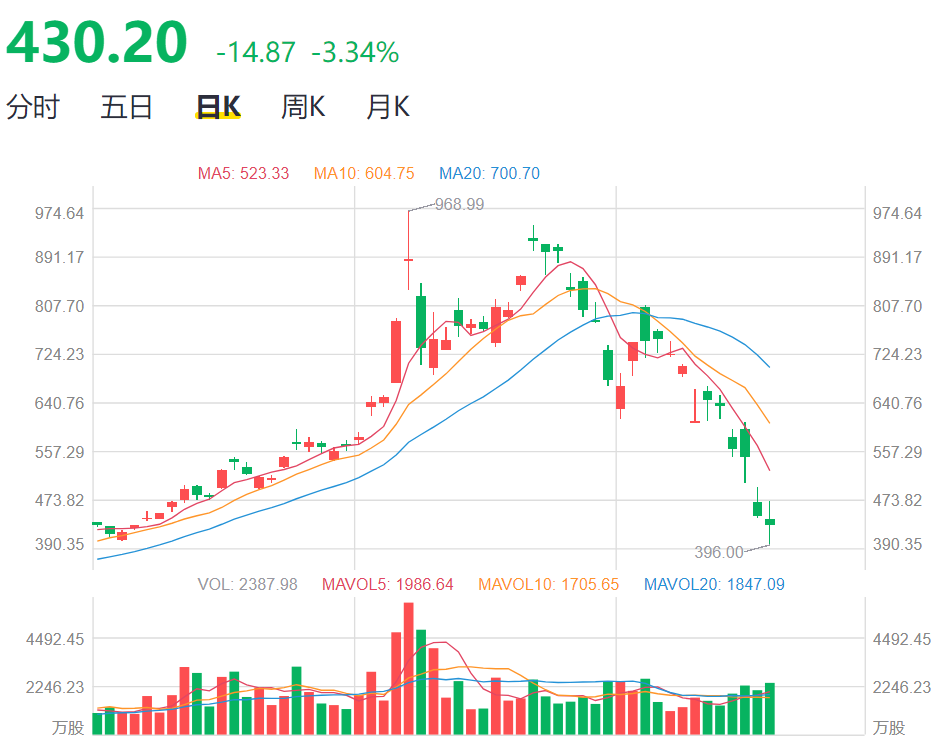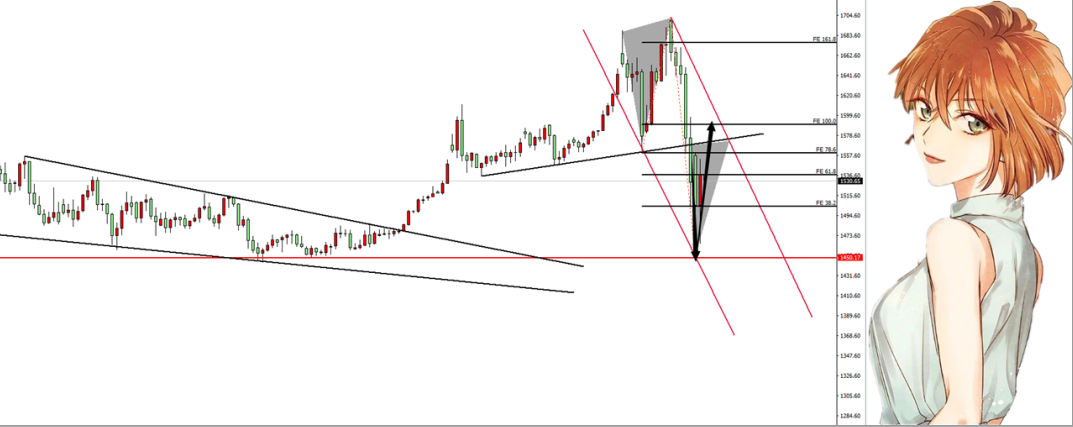500)this.width=500' align='center' hspace=10 vspace=10 rel='nofollow'/>500)this.width=500' align='center' hspace=10 vspace=10 rel='nofollow'/>500)this.width=500' align='center' hspace=10 vspace=10 rel='nofollow'/>500)this.width=500' align='center' hspace=10 vspace=10 rel='nofollow'/>500)this.width=500' align='center' hspace=10 vspace=10 rel='nofollow'/>500)this.width=500' align='center' hspace=10 vspace=10 rel='nofollow'/>500)this.width=500' align='center' hspace=10 vspace=10 rel='nofollow'/>

Part 2. 黄金抄底成功500)this.width=500' align='center' hspace=10 vspace=10 rel='nofollow'/>